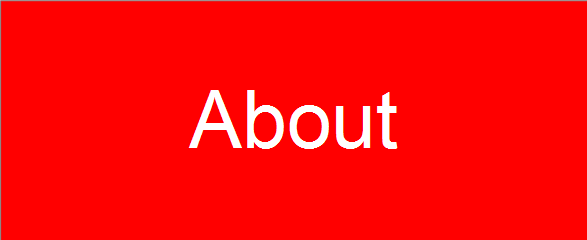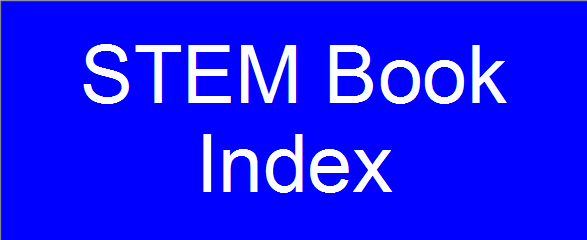• Chapter 1: Where PDEs Come From
• Section 1.1: What is a Partial Differential Equation?
• Section 1.2: First-Order Linear Equations
• Section 1.3: Flows, Vibrations, and Diffusions
• Section 1.4: Initial and Boundary Conditions
• Section 1.5: Well-Posed Problems
• Section 1.6: Types of Second-Order Equations

• Chapter 2: Waves and Diffusions
• Section 2.1: The Wave Equation
• Section 2.2: Causality and Energy
• Section 2.3: The Diffusion Equation
• Section 2.4: Diffusion on the Whole Line
• Section 2.5: Comparison of Waves and Diffusions

• Chapter 3: Reflections and Sources
• Section 3.1: Diffusion on the Half-Line
• Section 3.2: Reflections of Waves
• Section 3.3: Diffusion with a Source
• Section 3.4: Waves with a Source
• Section 3.5: Diffusion Revisited

• Chapter 4: Boundary Problems
• Section 4.1: Separation of Variables, The Dirichlet Condition
• Section 4.2: The Neumann Condition
• Section 4.3: The Robin Condition

• Chapter 5: Fourier Series
• Section 5.1: The Coefficients
• Section 5.2: Even, Odd, Periodic, and Complex Functions
• Section 5.3: Orthogonality and General Fourier Series
• Section 5.4: Completeness
• Section 5.5: Completeness and the Gibbs Phenomenon
• Section 5.6: Inhomogeneous Boundary Conditions
• Chapter 6: Harmonic Functions
• Section 6.1: Laplace's Equation
• Section 6.2: Rectangles and Cubes
• Section 6.3: Poisson's Formula
• Section 6.4: Circles, Wedges, and Annuli

• Chapter 7: Green's Identities and Green's Functions
• Section 7.1: Green's First Identity
• Section 7.2: Green's Second Identity
• Section 7.3: Green's Functions
• Section 7.4: Half-Space and Sphere

• Chapter 8: Computation of Solutions
• Section 8.1: Opportunities and Dangers
• Section 8.2: Approximations of Diffusions
• Section 8.3: Approximations of Waves
• Section 8.4: Approximations of Laplace's Equation
• Section 8.5: Finite Element Method

• Chapter 9: Waves in Space
• Section 9.1: Energy and Causality
• Section 9.2: The Wave Equation in Space-Time
• Section 9.3: Rays, Singularities, and Sources
• Section 9.4: The Diffusion and Schrodinger Equation
• Section 9.5: The Hydrogen Atom

• Chapter 10: Boundaries in the Plane and in Space
• Section 10.1: Fourier's Method, Revisited
• Section 10.2: Vibrations of a Drumhead
• Section 10.3: Solid Vibrations in a Ball
• Section 10.4: Nodes
• Section 10.5: Bessel Functions
• Section 10.6: Legendre Functions
• Section 10.7: Angular Momentum in Quantum Mechanics
• Chapter 11: General Eigenvalue Problems
• Section 11.1: The Eigenvalues Are Minima of the Potential Energy
• Section 11.2: Computation of Eigenvalues
• Section 11.3: Completeness
• Section 11.4: Symmetric Differential Operators
• Section 11.5: Completeness and Separation of Variables
• Section 11.6: Asymptotics of the Eigenvalues

• Chapter 12: Distributions and Transforms
• Section 12.1: Distributions
• Section 12.2: Green's Functions, Revisited
• Section 12.3: Fourier Transforms
• Section 12.4: Source Functions
• Section 12.5: Laplace Transform Techniques

• Chapter 13: PDE Problems from Physics
• Section 13.1: Electromagnetism
• Section 13.2: Fluids and Acoustics
• Section 13.3: Scattering
• Section 13.4: Continuous Spectrum
• Section 13.5: Equations of Elementary Particles

• Chapter 14: Nonlinear PDEs
• Section 14.1: Shock Waves
• Section 14.2: Solitons
• Section 14.3: Calculus of Variations
• Section 14.4: Bifurcation Theory
• Section 14.5: Water Waves

Exercises 1.1.1 - 1.2.4

Exercises 1.2.5 - 1.3.7

Exercises 1.3.8 - 1.5.5

Exercises 1.5.6 - 2.1.9

Exercises 2.1.10 - 2.3.8

Exercises 2.4.1 - 2.4.16

Exercises 2.4.17 - 3.2.8

Exercises 3.2.9 - 3.4.9

Exercises 3.4.10 - 4.2.2

Exercises 4.2.3 - 4.3.14

Exercises 4.3.15 - 5.1.11

Exercises 5.2.1 - 5.2.16

Exercises 5.2.17 - 5.3.15

Exercises 5.4.1 - 5.4.16

Exercises 5.4.17 - 5.5.12

Exercises 5.5.13 - 6.1.1

Exercises 6.1.2 - 6.2.5

Exercises 6.2.6 - 6.4.10

Exercises 6.4.11 - 7.2.2

Exercises 7.2.3 - 7.4.12

Exercises 7.4.13 - 8.1.3

Exercises 8.2.1 - 8.3.1

Exercises 8.3.2 - 8.4.6

Exercises 8.4.7 - 9.1.5

Exercises 9.1.6 - 9.2.13

Exercises 9.2.14 - 9.3.9

Exercises 9.4.1 - 10.1.7

Exercises 10.2.1 - 10.3.9

Exercises 10.3.10 - 10.5.8

Exercises 10.5.9 - 10.6.5

Exercises 10.6.6 - 11.2.3

Exercises 11.2.4 - 11.4.7

Exercises 11.5.1 - 12.1.4

Exercises 12.1.5 - 12.2.8

Exercises 12.2.9 - 12.4.2

Exercises 12.4.3 - 13.1.5

Exercises 13.1.6 - 13.5.2

Exercises 13.5.3 - 14.1.7

Exercises 14.1.8 - 14.2.11

Exercises 14.2.12 - 14.4.3

Exercises 14.4.4 - 14.5.7